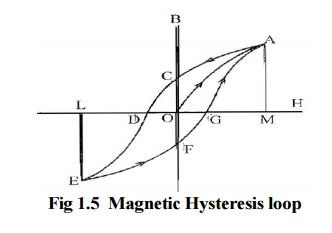Home | | Electrical Machines I | Properties of Magnetic Materials

# Properties of Magnetic Materials

1. Magnetic Hysteresis 2. Hysteresis Loop

Properties of Magnetic Materials

## 1. Magnetic Hysteresis

It may be defined as the lagging of magnetization or Induction flux density (B) behind the magnetizing force (H). It may also be defined as a quality of a magnetic substance due to which energy is dissipated in it on the reversal of its magnetism## 2. Hysteresis Loop

Let us take a un magnetized bar of iron AB and magnetize in by placing it within the magnetizing field of a solenoid (H). The Field can be increased or decreased by increasing or decreasing current through it. Let `H' be increased in step from zero up to a certain maximum value and the corresponding of induction flux density (B) is noted. If we plot the relation between H and B, a curve like OA, as shown in Figure, is obtained. The material becomes magnetically saturated at H = OM and has, at that time, a maximum flux density, established through it. If H is now decreased gradually (by decreasing solenoid current) flux density B will not decrease along AO (as might be expected) but will decrease less rapidly along AC. When it is Zero B is not zero, but has a definite value = OC. It means that on removing the magnetizing force H, the iron bar is not completely demagnetized. This value of B (=OC) is called the residual flux density.

To demagnetize the iron bar we have to apply the magnetizing force H in the reverse direction. When H is reversed by reversing current through the solenoid, then B is reduced to Zero at point D where H - OD. This value of H required to wipe off residual magnetism is known as coercive force and is a measure of the coercivity of materials i.e. its `tenacity' with which it holds on to its magnetism. After the magnetization has been reduced to zero value of H is further increased in the negative i.e. reverse direction, the iron bar again reaches a state of magnetic saturation represented by point E. By taking H back from its value corresponding to negative saturation (=OL) to its value for positive saturation (=OM), a similar curve EFGA is obtained. If we again start from G, the same curve GACDEFG is obtained once again. It is seen that B always lags behind H the two never attain zero value simultaneously. This lagging of B, behind H is given the name Hysteresis' which literally means `to lag behind.' The closed Loop ACDEFGA, which is obtained when iron bar is taken through one complete cycle of reversal of magnetization, is known as Hysteresis loop.

Study Material, Lecturing Notes, Assignment, Reference, Wiki description explanation, brief detail
Electrical machines : Magnetic Circuits and Magnetic Materials : Properties of Magnetic Materials |